# Floor Node

Access: NodesMathFloor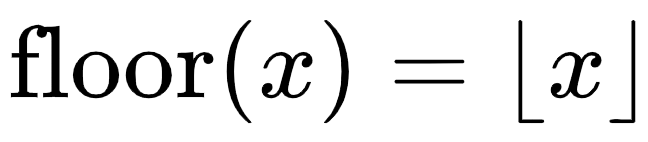The Floor node rounds down values to the nearest whole number or integer.

Note:  The Floor node is similar to the Ceil node operation, except instead of rounding up to the nearest whole number, Floor rounds down. For more information, see the Ceil node.

 Input Output 3.75, 5.08, 2.49, 1.0 3, 5, 2, 1 5.0, 6.5, 7.2, 1.0 5, 6, 7, 1 0.0, 0.3, 0.7, 0.0 0, 0, 0, 0

# Floor Node Inputs

 Input The input values to be rounded down to the nearest integer.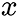# Floor Node Outputs

 Output The input after rounding down to the nearest whole number.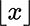# Floor Node Workflow Example

The Floor node can be used to create a simple posterize effect from a texture color input. To define the number of levels in the posterize effect, you can use the Multiply and Divide nodes, in the following way, where the Multiply and Divide B inputs are the number of levels you would like in the posterize effect: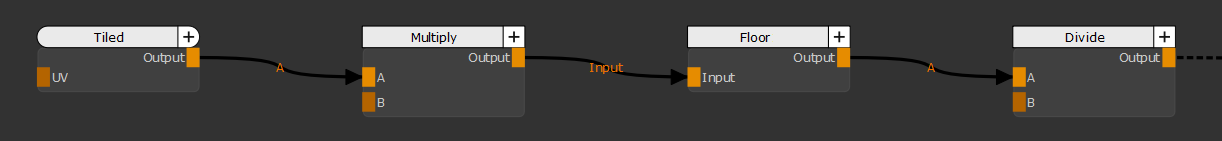Workflow to Create a Posterize Effect using a Floor Node

Note:  The Floor node produces a similar posterize effect to the Ceil node but because it rounds the input values down, the resulting colors will appear darker.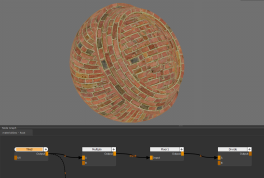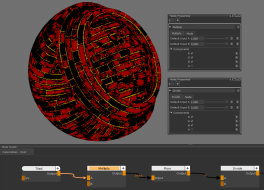Input Texture Multiply and Divide B input of 3. Resulting in 3 Posterize Levels.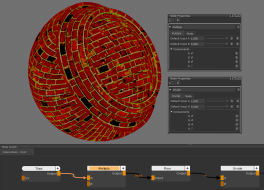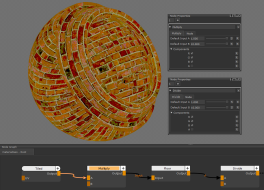Multiply and Divide B input of 5. Resulting in 5 Posterize Levels. Multiply and Divide B input of 10. Resulting in 10 Posterize Levels.